# Uniform Distribution

## What is a Uniform Distribution?

A Uniform Distribution is a distribution in which there equal probabilities across all the values in the set. Also known as the continuous uniform distribution or rectangular distribution, a uniform distribution is bounded by two main parameters,

a and b, the minimum and maximum values.

## How Does a Uniform Distribution Work?

The Uniform Distribution of continuous variables have constant probability. This can be displayed mathematically with the functions for a continuous probability density function, and cumulative distribution function.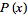=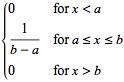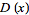=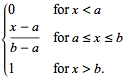The probability density function and continuous distribution function can be displayed graphically as well. Note in the probability density function, all values between the minimum and maximum values are equal in probability.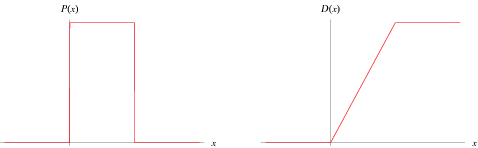Source

### Example of Uniform Distribution

Imagine wanting to display the probability that a coin toss will land on the heads. The probability of the coin landing on one side or the other is always .5 or 50%, regardless of how many times one tosses the coin. Whether tossing for the first time, or tenth, the probability of landing on one specific side will remain at 50%. The distribution of these probabilities would graphically mirror that of a uniform distribution.